# Twisted One Reasoning Ability Quiz for All Banking Exams- 30th April

Directions (1-5): Study the following information carefully and answer the questions given below:
Six Horse i.e. P, Q, R, S, T and U are standing in a row facing north at a distance which is a successive multiple of 6 in an increasing order from the left to right.
Horse S is standing second from one of the extreme end. Only two horses stand between the S and T. Two horses stand between R and P who does not stand adjacent to S. U stands right of Q, who does not stand next to P. Neither R nor S is not an immediate neighbour of Q. Total distance between the horse P and Horse S is 54. Now, horse U is moving towards North direction and after going 10m, it turns to right and goes 15m to reach at point Y. Horse R is moving towards South direction and after going 15m it takes right turn and goes 51m to reach at point Z.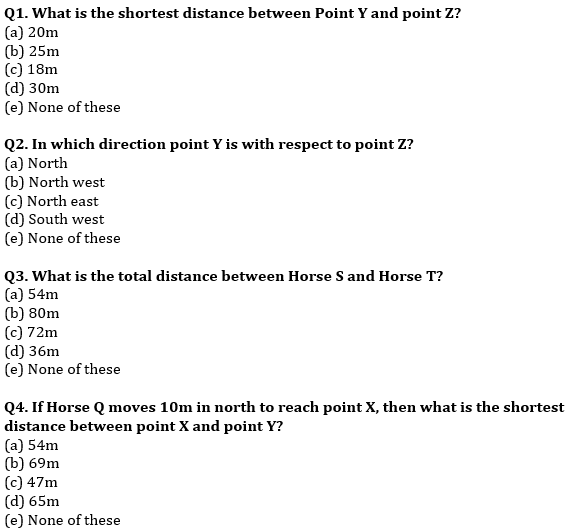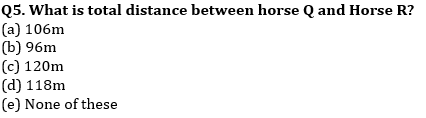Directions (6-10): In the following questions, the symbols #, %, \$, @ and © are used with the following meaning as illustrated below.
‘P # Q’ means ‘P is not smaller than Q’.
‘P % Q’ means ‘P is neither smaller than nor equal to Q’.
‘P \$ Q’ means ‘P is neither greater than nor equal to Q’.
‘P @ Q’ means ‘P is neither greater than nor smaller than Q’.
‘P © Q’ means ‘P is not greater than Q’.
Now, in each of the following questions assuming the given statements to be true, find which of the three Conclusions I, II and III given below them is/are definitely true and give your answer accordingly.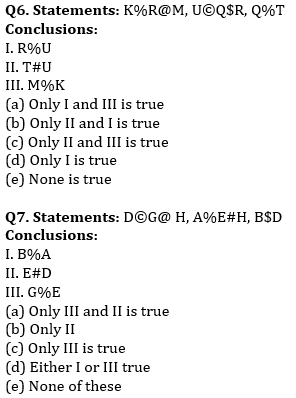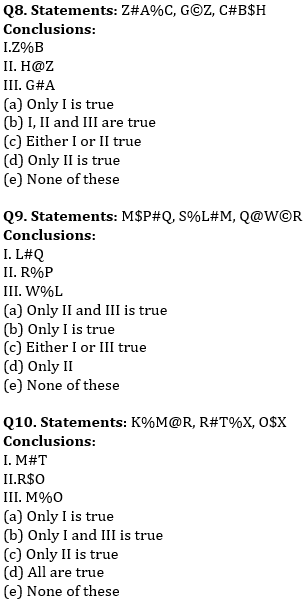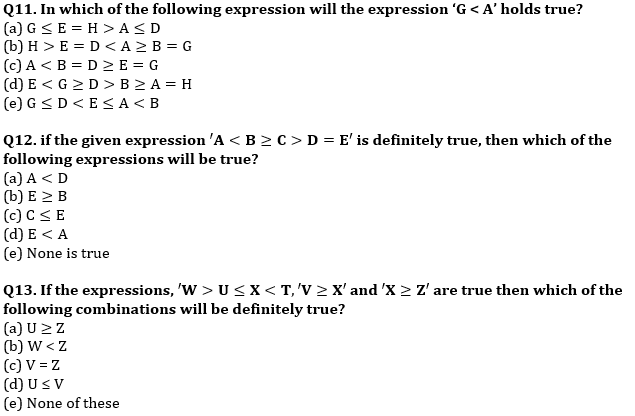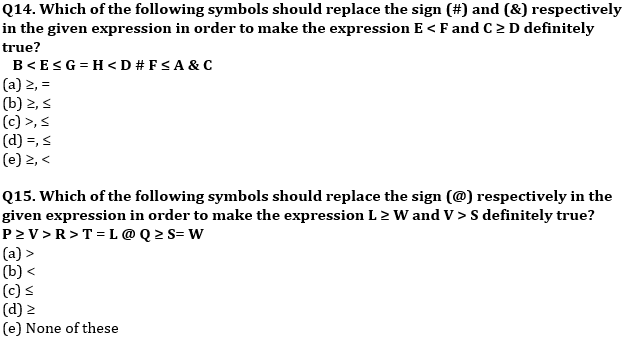Practice More Questions of Reasoning for Competitive Exams:

Solutions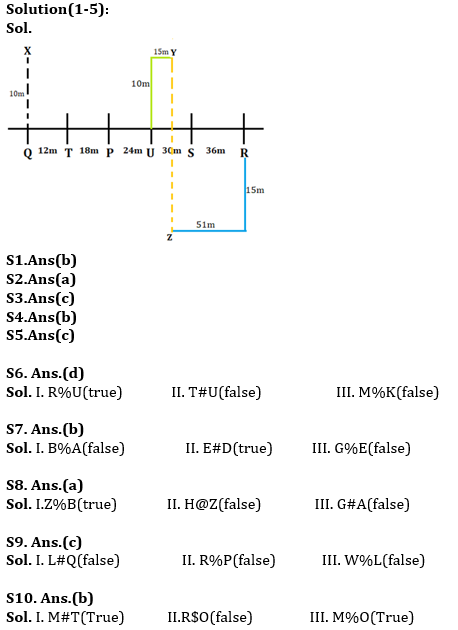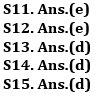×

Thank You, Your details have been submitted we will get back to you.
×
OR

×
OR